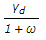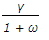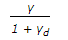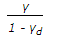# Civil Engineering - Soil Mechanics and Foundation Engineering

Exercise : Soil Mechanics and Foundation Engineering - Section 3
1.
The vane shear test is used for the in-situ determination of the undrained strength of the intact fully saturated
sands
clays
gravels
highly organic soils.
Explanation:
No answer description is available. Let's discuss.

2.
Fundamental relationship between dry density (γd), bulk density (γ) and water content (ω), is :
γ =γd =ω =ω =Explanation:
No answer description is available. Let's discuss.

3.
The specific gravity of quartz, is
2.65
2.72
2.85
2.90
Explanation:
No answer description is available. Let's discuss.

4.
If the cohesive force, (c), is 1.5 t/m2, the density ) of the soil is 2.0 t/m3, factor of safety (F) is 1.5 and stability factor (Sn) is 0.05, the safe height of the Â§lope, is
5 metres
8 metres
10 metres
12 metres